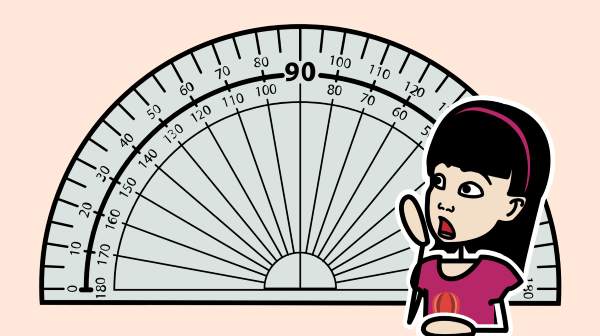×

## When Would You Like to Meet?The basics of angles are so important for students to understand! Angles play a critical role in geometry and are the foundation for trigonometry and geometry. Students should eventually be able to read a protractor, but even just understanding if an angle is big (obtuse or reflex) or small (acute) makes a difference in elementary geometry. The following activities are a great way to provide visual aids to students and help them understand how different angles affect polygons.

## Types of Angles Background

When learning and classifying polygons, one of the things we look at is the number of angles or the size of interior angles. Understanding the rotation of angles will certainly be relevant in trigonometry with sine, cosine, and tangent, but that is a long way off for students just being introduced to angles. On Storyboard That, students can construct physical representations of polygons using various items for sides, and they will see that the size of the angle directly affects the side length of triangles and other polygons, connecting to types of triangles, the Pythagorean Theorem, perimeter, and more.

We can identify the type of polygon by the number of angles too! Quadrilateral also indicates quadrangle. “Four sides” means four angles. “Six sides” means six angles. “Twenty-seven sides” means twenty-seven angles. Irregular polygons may even have reflex angles that students wouldn’t normally be able to identify because they are so big or so strange. Angles may seem straightforward, but they are actually very involved and crucial to geometry and beyond.

For additional practice with angles, consider making worksheets! With the help of worksheet templates, create tailored content for your students. Students can practice measuring angles, matching definitions and types of angles, and even create worksheets for their classmates as a way to take ownership over their education and help each other.